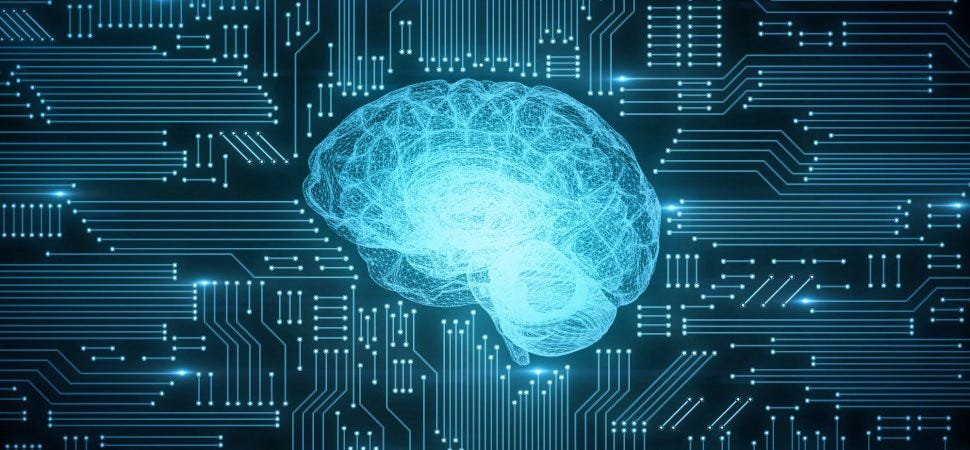# A Beginner’s Guide to Machine Learning

Original article can be found here (source): Artificial Intelligence on Medium# A Beginner’s Guide to Machine Learning

Have you ever wondered how Netflix chooses what movies to suggest, how Siri can respond to your commands, or how autonomous vehicles are able to navigate the roads? Well, it’s all thanks to the field of study known as artificial intelligence–or AI for short.

But what exactly is the field of AI, and what is it concerned with?

Well, AI simply refers to the simulation of human processes by machines. In other words, it is essentially the quest to build machines that can reason, learn, and act intelligently.

Pretty straightforward, right?

But AI is an umbrella term for many more specific subcategories or “branches” along with many manifestations.

Let’s take a look at the most common branch of AI: machine learning.

# Machine Learning

Machine Learning is using data to answer questions.

However, before a machine can turn inputted data into answers, it must first be trained. This process is known as data mining, and there are 3 different ways to do it:

## 1. Unsupervised Learning

Unsupervised learning occurs when inputted data is not labelled. The machine is forced to take in the data and discover information about the data on its own. The machine does this by modelling any hidden patterns or underlying structures in the given input data.

An example of this is cluster analysis. Visually, it looks like the graph on the right. The inputted data points have been separated into 3 clusters based on the machine’s chosen similarities. This algorithm is known as K-Means.

Another application of this is anomaly detection. For example, in the data provided above, there is a green data point at the top of the black cluster. This point serves as an outlier and could potentially be a fraudulent data point.

A problem that may arise when trying to use unsupervised learning is that there are many different angles a machine can sort inputted data. Sometimes, the machine can be unpredictable and sort the data in a way you don’t want.

## 2. Supervised Learning

Supervised learning, on the other hand, occurs when inputted data is labelled. Labelled data is inputted data that already has corresponding outputs attached to each data point. The machine then uses labelled data to learn and train itself.

After training itself with large amounts of data, the machine’s algorithm would then be able to accurately predict what the output of an unlabelled data point would be based on which labelled data point the unlabelled data point best fits with.

Two useful examples of supervised learning are image classification and regression.

Image classification is done through convolutional neural networks (CNNs). CNNs work by first taking in a large number of photos of a specific object — like the car in the illustration above. From this inputted data, it assigns importance (weightings) to various aspects of the object in order to differentiate images of the object from images of other objects. When another image is inputted, it checks the weightings of the aspects of that image and compares it to that of the learned ones in order to classify the inputted image.

Another, much simpler, example of supervised learning is regression which is used to predict continuous values. An example of this is a linear regression which is shown to the left. It models a line that predicts the y-value of a data point based on its x-value.

A problem that may arise when trying to use supervised learning is the idea of overfitting. If the machine puts too much emphasis on the accuracy of the placement of labelled data and new data, the machine might break.

## 3. Reinforcement Learning

Reinforcement learning occurs when a machine has a goal that it is trying to achieve and does so through trial-and-error. The machine uses the idea of maximizing its cumulative rewards for each trial. It is able to increase its rewards by utilizing feedback from previous failed attempts until it finally reaches the best outcome and achieves its goal.

One way reinforcement learning has been used is when a machine is trying to beat a video game — Geometry Dash, for example. The machine will, at first, have no idea what to do. But, after many iterations, it will slowly figure out at what times it needs to jump to complete the level.

But how does deep learning come into all this?

# Deep Learning

Deep learning is the branch of machine learning where self-teaching systems use existing data to make predictions about new data through patterns found by algorithms.

Deep learning machines can be trained either through unsupervised learning or supervised learning, and they work by using artificial neural networks that mimic the neurons in the human brain.

The neural networks that are used have three layers: the input layer, the hidden layer, and the output layer.

1. The Input Layer is simply where initial data is brought into the system.
2. The Hidden Layer is located in the middle of the input layer and the output layer, and it is where all the “deep learning” actually occurs. It works by performing learnable computations on the weighted inputs to produce a net input which is then applied with a non-linear activation function to produce the final output.
3. The Output Layer is what produces the results for the given input data.

The difference between a traditional machine learning neural network and a deep learning neural network is the complexity of the hidden layer. Deep learning neural networks have multiple hidden layers allowing the algorithm to model non-linear relationships.

Now that we know what deep learning is, let’s take a look at a useful application.